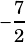Hi! May you please explain number 8 from the practice questions in the “lines” chapter? I understand that A is the correct answer after looking at the table values, but I don’t understand it from the equation itself. Thanks!

Sure. You’re given the equation, but because this is the lines chapter, let’s put it in slope-intercept form.We’ve got a slope ofthere, which means the dependent variable (, in this case) decreases by 7 every time the independent variable () increases by 2.ar123 says: